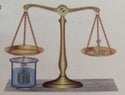# Relationship between buoyancy Force and the gravitational force between two bodies?

Callmelucky
Homework Statement:
Picture below. Both bodies are made of same material but the body placed in water had 2.4 times greater mass. What is the relationship between buoyancy Force and gravitational force between body in water and body in air?
Relevant Equations:
F(buoyancy) = density(of fluid) * g * Volume(of object), F(gravitational) = m * g
Picture below. Both bodies are made of same material but the body placed in water had 2.4 times greater mass. What is the relationship between buoyancy Force and gravitational force between body in water and body in air?

I have no clue how to solve this.
I know that body with greater mass has 2.4 times greater buoyancy Force, 2.4 times greater mass and gr. force, 2.4 larger volume. But I don't know how to solve the problem.

The worst thing is that I only need to answer if F(buoyancy) of body in water is <, =, > than gravitational force of body in air or if there is not enough data to answer the question.
Answer at the end of textbook is F(buoyancy) of body in water > F(gravitational) of body in air.

if some can please explain I'd be very grateful.
Thank you.

#### Attachments

•IMG_20230207_035319_edit_1066088544897222.jpg
34.5 KB · Views: 15

Homework Helper
What can you infer from the fact that the scale is in equilibrium? What is the relationship between the net forces acting on the two bodies?

•Callmelucky
Callmelucky
What can you infer from the fact that the scale is in equilibrium? What is the relationship between the net forces acting on the two bodies?
well, I guess that Fb+Fg1 = Fg2, but I still don't get it.

Homework Helper
Gold Member
well, I guess that Fb+Fg1 = Fg2
Don't guess. Draw two free body diagrams, one for each mass, and show all the forces acting on the mass in each diagram.

•Callmelucky
Callmelucky
Don't guess. Draw two free body diagrams, one for each mass, and show all the forces acting on the mass in each diagram.
🤦‍♂️🙆‍♂️ How stupid I am. I got it. Thank you.
I didn't solve enough problems with drawing forces, so I don't have that habit.
I will have to fix that.

Last edited:
•kuruman
•